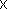!! used as default html header if there is none in the selected theme. OEF Powers

# OEF Powers --- Introduction ---

This module currently contains 19 exercises on powers of a number, powers of 10, scientific notation .

### Decimal numbers and powers of 10

Write the number in two ways :
• scientific notation
=10
• as with integer and not multiple of 10 :
=10

### Definition of scientific notation

Write the following number using scientific notation:

### Definition of a power of 10

Determine the value of the number
You will write the answer as an integer or decimal number.

### Definition of power, positive integer exponent

Determine the value of the number

### Definition of power, relative integer exponent

Determine the value of the number
.
Give the result as an integer or an irreducible fraction.

### Bounds of decimal numbers

Give bounds of the number by consecutive powers of 10.
10 < 10

### Operation 1

Complete the equality so that it becomes true:
Give the result in the form .

### Operation 2

Complete the equality so that it becomes true:
Give the result in the form .

### Order of magnitude

Determine an order of magnitude for the number , as shown in class:
If necessary, the exponent in the power of 10 can be zero..

### Power of a number

Write as a power of an integer (with the largest possible power):
=

### Powers of 10

Write the number as = 10

### Powers

The number is . Write it as a product of powers :
= == -= -\

:

:

:
• =
• =
• =

:
• =
• =
• =

:
• =
• =
• =

### Table of powers

Complete the table:

### Table of powers II

Complete the table:
In order to access WIMS services, you need a browser supporting forms. In order to test the browser you are using, please type the word wims here: and press Enter''.